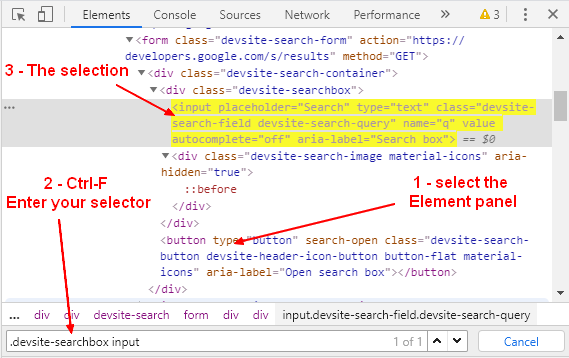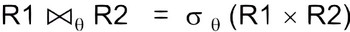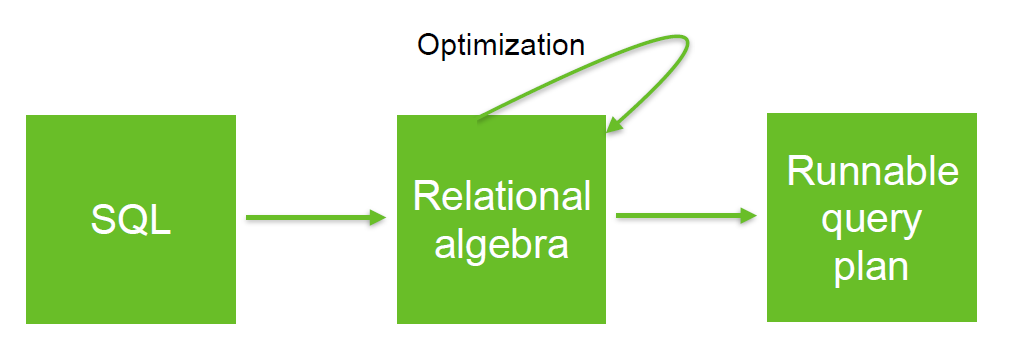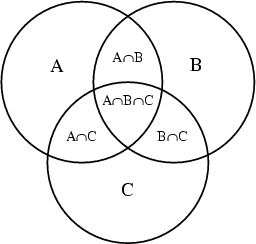# Language - Equality

The Equality operators model the Equivalence relation:

• equality
• is parallel to (for affine spaces)
• is in bijection with
• isomorphy

The Equality operators are a subset of the comparison operator.

The = symbol was designed to be two lines of visibly equal length.

## Usage

The equality operators as comparison operator are used in predicate (condition) expression in order to:

## Operator

Equality operator

Operation Description
== loose-equals, same value, two different Type
such as '2' in number of '2' as string are loose-equals
=== strict-equals, same value, same type
!= loose not-equals
<> loose not-equals (should be deprecated)
!== strict not-equals
is object identity
is not negated object identity

The operator = is generally not a comparison operator but an assignment operator

## Mathematics

• Approximately equal

$$a \approx b$$

## Evil in loop predicate

The equality operator is evil in a loop predicate.

The common error is to find a == in the code where there should have been a >= or a <=

See:

Discover MoreFunction - Order

Ordering is the process of finding the position of an element of ordinal data type in a sequence. Order and Equivalence are a special kind of binary relation (ie relation between two entities) With the...How to select elements based on attribute ? (with the css selector API)

This page is about selector expressions that select elements based on attribute. * An ID selector and a class selector (ie selects element with ID “IdName” and the class “className”) *...
Javascript - Equality

Loss Equality A loss equality will not check the type of the value and will perform a coercion before checking the equality. A strict equality will also check the type of the value.
Javascript - Object Equality

This page is talking Equality for an object in the sense, not a primitive. For a comparison between object, both == and === object equality operator will simply check whether the references match....Language - Assignment

An assignment is an expression that gives a value to a variable. assignmentstatementexpression assignmentstatementassignment In its simplest form, an assignment statement has a variable on the...Ordinal Data - Comparison Operator (Order, Equality)

A comparison operator is a class of operators that model: a order or a equivalence relationship A comparison operator test the order relationship between two operands and returns a Boolean. The...Relational Algebra - Theta-join

A theta-join is a difficult/complex join where the condition is not a equality. Example: Band join or range join. A theta is a join that links tables based on a relationship other than the equality...Relational Operator - Equi-joins

An Equi-join is a join where the condition (predicate) is an equality. theta join An equi-join links two relations (tables, ...) on common values in a column in relation 1 with a column in relation...Set theory - Equality

in set theory The equality relationship indicates that two sets are equal if they contain exactly the same elements. (There is no order among elements of a set.) Two sets are equal if they contain...